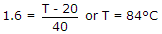# Electronics and Communication Engineering - Power Electronics

### Exercise :: Power Electronics - Section 3

1.

In a dual converter both converters work as rectifiers.

 A. True B. False

Explanation:

One operators as rectifier and the other as inverter.

2.

A single phase full wave regulator feeds R-L load. The best gating signal is

 A. short duration pulses B. long duration pulses C. pulse train D. either (a) or (b)

Explanation:

To ensure proper triggering pulse train in necessary because load is R - L.

3.

A 3 phase induction motor is fed by a 3 phase ac regulator to change the stator voltage. The variation in speed will be more if

 A. load torque is constant B. load torque increases with increase in speed C. load torque decreases with increase in speed D. either (a) or (b)

Explanation:

If load torque is constant, the change in speed is very small.

4.

A cycloconverter cannot give a frequency higher than input frequency.

 A. True B. False

Explanation:

Step up cycloconverter can give a frequency higher than input frequency.

5.

A thyristor has internal power dissipation of 40W and is operated at an ambient temperature of 20°C. If thermal resistance is 1.6° C/W, the junction temperature is

 A. 114°C B. 164°C C. 94° C D. 84° C.